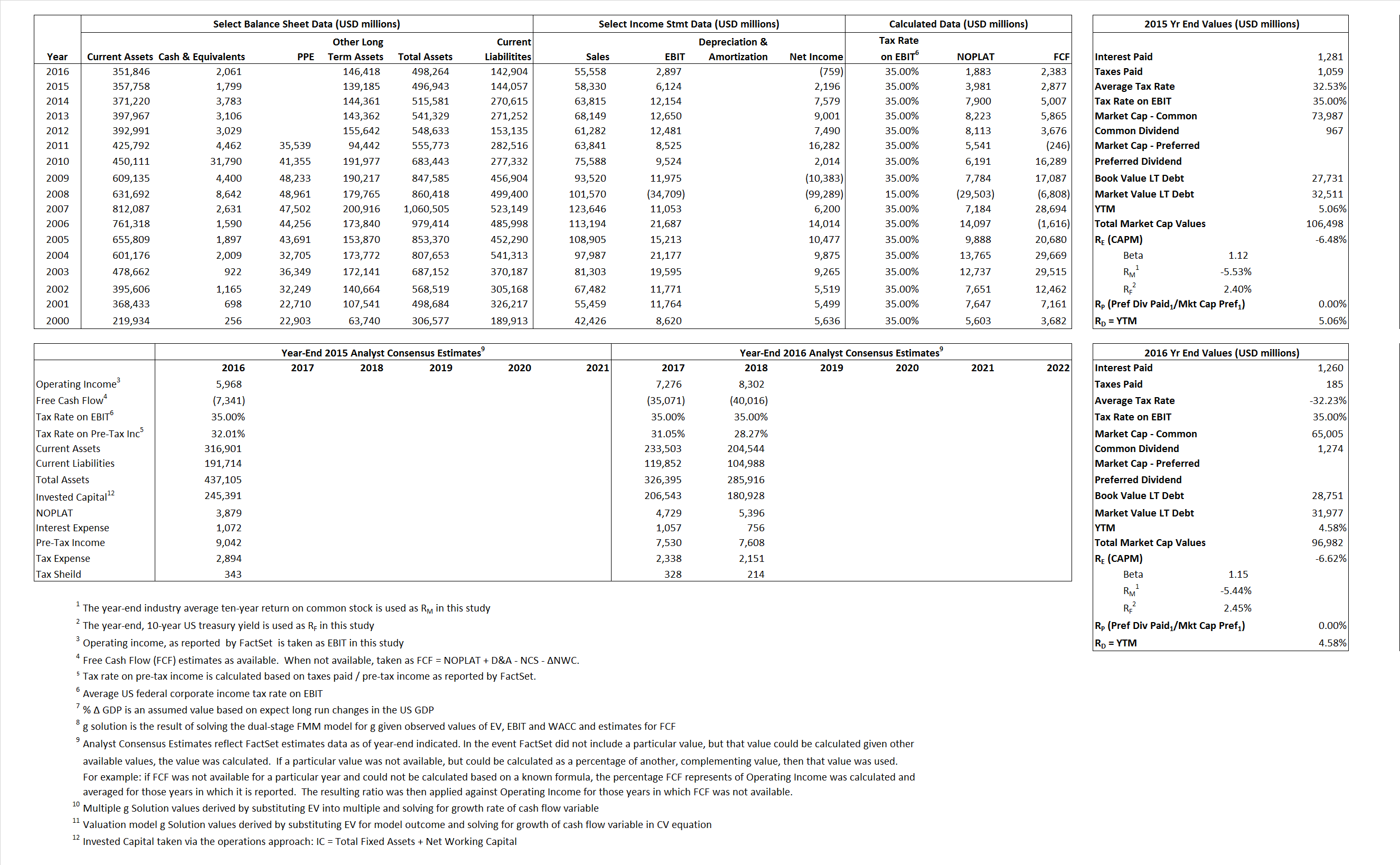# American International Group

## Analyst Listing

The following analysts provide coverage for the subject firm as of May 2016:

 Broker Analyst Analyst Email Wells Fargo Securities Elyse Greenspan elyse.greenspan@wellsfargo.com FBR Capital Markets & Co Randy Binner rbinner@fbr.com RBC Capital Markets Mark Dwelle mark.dwelle@rbccm.com Bernstein Research Josh Stirling josh.stirling@bernstein.com Atlantic Equities John Heagerty j.heagerty@atlantic-equities.com Piper Jaffray John Nadel john.m.nadel@pjc.com Sandler O’Neill & Partners Paul Newsome pnewsome@sandleroneill.com Janney Montgomery Scott Larry Greenberg lgreenber@janney.com Keefe Bruyette & Woods Meyer Shields mshields@kbw.com William Blair Adam Klauber aklauber@williamblair.com BMO Capital Markets Charles J. Sebaski charles.sebaski@bmo.com Deutsche Bank Research Joshua Shanker joshua.shanker@db.com Credit Suisse Ryan Tunis ryan.tunis@credit-suisse.com

## Primary Input Data## Derived Input Data

### Equational Form

Net Operating Profit Less Adjusted Taxes NOPLAT 3,981  1,883$NOPLAT\, =\, EBIT\, x\, (1 \,-\, Avg \,\,Tax\,\, Rate\,\, on\,\, EBIT)$
Free Cash Flow FCF 2,877 2,383$FCF\,=NOPLAT\,+\,Non-Cash\,Expenses-\Delta NWC\,-\,NCS$
Tax Shield TS 417 (406)$TS\,=\,Interest\,\,Paid\,\,x\,\, Avg \,\,Tax\,\,Rate\,\, on\,\, Pre-Tax\,\, Income$
Invested Capital IC 352,886 355,360$IC\,=\,Fixed\,\,Operating\,\,Assets\,\,+\,\,Net\,\, Working\,\, Capital$
Return on Invested Capital ROIC 1.13% 0.53%$ROIC\,=\,\frac { NOPLAT }{ IC }$
Net Investment NetInv 107,920 2,474$NetInv\,=\,{ {IC}_{1}}-{{IC}_{0}}+Depreciation$
Investment Rate IR 2,711.15% 131.38%$IR\,=\,\frac {NetInv}{NOPLAT}$
Weighted Average Cost of Capital
WACCMarket -3.46% -2.44%$WACC\,=\,\frac { E }{ V } { R }_{ E }\,+\,\frac { P }{ V } { R }_{ P }\,+\,\frac { D }{ V } { R }_{ D }\left( 1- Avg\,\, Tax\,\,Rate\,\,on\,\,Pre-Tax\,\,Income \right)$
WACCBook  7.90%  7.80%
Enterprise value
EVMarket 104,699  94,921$EV\,=\,Market\,\,Cap\,\,Equity\,+\,\,Long\,\,Term\,\,Debt\,-\,Cash$
EVBook  99,919  91,695
Long-Run Growth
g = IR x ROIC
30.58%  0.70% Long-run growth rates of the income variable are used in the Continuing Value portion of the valuation models.
g = %$\Delta$ GDP  2.50%  2.50%
Margin from Operations M  10.50%  5.21%$M\,\,=\,\,\frac{EBIT}{SALES}$
Depreciation/Amortization Rate D 0.00%  0.00%$D\,\,=\,\,\frac{D+A}{EBITDA}$

## Valuation Multiple Outcomes

The outcomes presented in this study are the result of original input data, derived data, and synthesized inputs.

### model g solution

12/31/2015 12/31/2016 12/31/2015 12/31/2016 12/31/2015 12/31/2016

EV/SALES$\frac {EV}{Sales} \,= \,\frac{ROIC\, -\, g}{ROIC\,(WACC\,-\,g)}\,(1\,-\,T)\,(M)$

1.79  1.71  3.06%  1.61%  3.16%  1.54%

EV/EBITDA$\frac {EV}{EBITDA} \,= \,\frac{ROIC\, -\, g}{ROIC\,(WACC\,-\,g)}\,(1\,-\,T)\,(1\,-\,D)$

17.10

32.77 3.06% 1.61% 3.16% 1.54%

EV/NOPLAT$\frac {EV}{NOPLAT} \,= \,\frac{ROIC\, -\, g}{ROIC\,(WACC\,-\,g)}$

26.30 50.41 3.06% 1.61% 3.16% 1.54%

EV/FCFOPS$\frac {EV}{FCF_{OPS}} \,= \,\frac{ROIC\, -\, g}{ROIC\,(WACC\,-\,g)}\,(1\,-\,T)$

36.39

39.83 3.06% 1.61% 3.16% 1.54%

EV/EBIT$\frac {EV}{EBIT} \,= \,\frac{ROIC\, -\, g}{ROIC\,(WACC\,-\,g)}\,(1\,-\,T)$

17.10

32.77 3.06% 1.61% 3.16% 1.54%

EV/IC$\frac {EV}{IC} \,= \,\frac{ROIC\, -\, g}{WACC\,-\,g}$

0.30

0.27 3.06%  1.61% 3.16% 1.54%